# What is CnH2n 2

Not only Lilly in GZSZ desperately in her Abitur preparations at the number combinations that describe the general sum formulas of the homologous series of hydrocarbons. It is now called CnH2n or CnH2n + 2, and where does the 2 come from anyway? It seems even more complicated with them Alkenes and only then with the Alkynes to become. And then what about them Isomers?

You can practice that. We want to show that here. First a note: Here you can also work nicely with molecular models - e.g. B. with the fabulous stick-ball model kit from CVK.

1. Alkanes
First we write down the structural formula (= constitutional formula) of butane: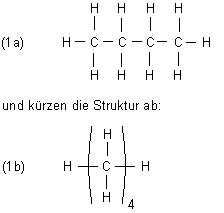We see that all four carbon atoms each have two hydrogen atoms. Be n the number of carbon atoms, then the number of hydrogen atoms is first 2n. The two terminal carbon atoms, however, each have one more hydrogen atom. (So ​​that's where the 2 comes from - which Lilly couldn't explain ...) So:

(1c) General empirical formula of the alkanes = CnH2n + 2

For butane is n = 4. This means that its molecular formula is C4H2 x 4 + 2 = C4H10.

We then practice that, starting with methane (n = 1).

2. Alkenes
We choose butene-1 as the test substance.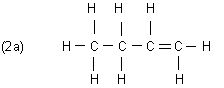We see that almost all C atoms have a different number of H atoms (exactly: 1, 2, 2, 3). Counting shows that in alkenes the number of C atoms is exactly half as large as that of H atoms. Hence:

(2b) General empirical formula of the alkenes = CnH2n

For butene is n = 4. This means that its molecular formula is C4H8.

To practice we have to go with you n = 2 or begin with ethene.

(The general formula for alkenes also applies to Cycloalkaneshow to z. B. can easily make clear on the basis of the cyclohexane.)

3. Alkynes
Here is the constitutional formula of butyne-1: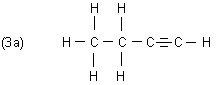We see that all C atoms here really have a different number of H atoms (exactly: 0, 1, 2, 3). Again, we count down for the sake of simplicity. Is n the number of carbon atoms is the number of hydrogen atoms 2n-2.

(3b) General empirical formula of the alkynes = CnH2n-2

For butyne is with n = 4 the empirical formula C4H6.

We first draw the structural formulas of the two positional or constitutional isomers of butane.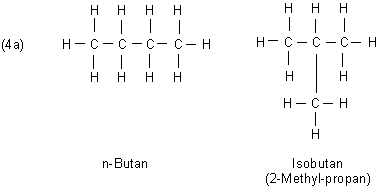Counting results in for both Molecular formulas C4H10.

The same applies to the three isomers of Butene: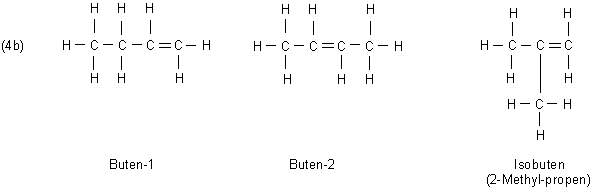The molecular formula all Butene isomer is C.4H8.

Of Butyne there are two isomers: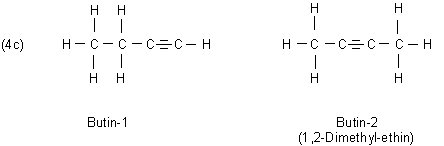The molecular formula of both Isomer of butyne is C4H6.

We learn from this:

The general empirical formula is the same for positional or constitutional isomers.

What if you still can't remember the expressions for the general structural formulas?
Then the following helps: You are certainly familiar with the hydrocarbons n = 2: Ethane, ethene, ethine. With these you can easily derive the general sum formulas.

 Hydrocarbon group Fabric withn = 2 Semi-structuralformula GeneralMolecular formula Alkanes Ethane CH3-CH3 C.nH2n + 2 Alkenes Ethene CH2= CH2 C.nH2n Alkynes Ethine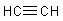C.nH2n-2

R diger flower

More tips of the month